Topics in

P R E C A L C U L U S

12

# Synthetic Division by x − a

The remainder theorem

IN ARITHMETIC we write, for example,or,

 47 5 =  9 + 25 .

Equivalently,

47 = 9· 5 + 2

5 is called the divisor,  47 is the dividend,  9 is the quotient,  and 2 is the remainder.

 Dividend Divisor =  Quotient  + Remainder  Divisor

Or,

Dividend  =  Quotient· Divisor  +  Remainder.

In algebra, if we divide a polynomial P(x) by a polynomial D(x) (where the degree of D is less than the degree of P), we would find

P(x) = Q(x)· D(x) + R(x).

P(x) is the dividend,  Q(x) is the quotient, and R(x) is the remainder.

For example, if, by long division, we divided

x3 − 5x2 + 3 x − 7   by   x − 2,

we would find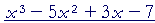x − 2 = x2 − 3x − 3 − 13  x − 2

Or,= (x2 − 3x − 3)(x − 2) − 13.

x3 − 5x2 + 3x − 7 is the dividend,  x2 − 3x −3  is the quotient,  and −13 is the remainder.

Here is how to do this problem by synthetic division.

First, to use synthetic division, the divisor must be of the first degree and must have the form  x − a.  In this example, the divisor is  x − 2, with a = 2.

Here again is the problem:x − 2

Proceed as follows:

1.  Write the coefficients of the dividend:

1 − 5 + 3 − 7

2.  Put a, in this case 2, in a box to the right, leave a space, and draw a
2.  line:3.  Bring down the leading coefficient (1), multiply it with a  (2), and
3.  write that product (1·  2) in the second column:5.  Repeat the process.   −3· 2 = −6.  And so on, until all the coefficients
5.  have been exhausted.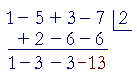The first three numbers, 1 − 3 − 3, are the coefficients of the quotient, and the final number, −13, is the remainder.

We have

x3 − 5x2 + 3 x − 7 = (x2 − 3x −3)(x − 2) − 13.

Example 1.   Use synthetic division to divide

2x5 + 3x4 + 25x2 − 1  by  x + 3.

Solution.   There are a couple of points here.  First, we must account for all six coefficients of the general form.

2 + 3 + 0 + 25 + 0 − 1

The coefficient of x3 is 0, as is the coefficient of x.

Next, the divisor is x + 3.  But the divisor must have the form  xa.

x + 3 = x − (−3).

Therefore, a = −3.

Here is the synthetic division:This tells usx + 3 = 2x4 − 3x3 + 9x2 − 2x + 6 − 19  x + 3

Or,

 2x5 + 3x4 + 25x2 − 1 = (2x4 − 3x3 + 9x2 − 2x + 6)(x + 3) − 19. Dividend = Quotient· Divisor  +  Remainder.

Note:  The degree of the quotient is one less than the degree of the dividend.  And the degree of the remainder is less than the degree of the divisor, x + 3, which in this case is 1.  The remainder therefore is of degree 0, which is a number.

In general, if we divide a polynomial of degree n by a polynomial of degree 1, then the degree of the quotient will be n − 1.  And the remainder will be a number.

Problem 1.   Use synthetic division to divide

x3 − 8x2 + x + 2   by  x − 7.

P(x) = Q(x)· D(x) + R.x3 − 8x2 + x + 2 = (x2x − 6)(x − 7) − 40

The remainder theorem

The value of a polynomial P(x) at x = a,

P(a),

is equal to the remainder upon dividing P(x)
by xa.

That is, when

P(x) = Q(x)(xa) + R,

where Q(x) is the quotient and R is the remainder, then

P(a) = R.

For,

 P(a) = Q(a)(a − a) + R = Q(a)· 0 + R = 0 + R = R.

Example 2.   Let  f(x) = x3 − 3x2 − 13x + 15.

We will use synthetic division to divide f(x) by x + 4.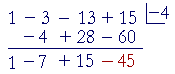Now, what does the remainder theorem tell us?

The value of f(x) at x = −4, is equal to the remainder:

f(−4) = −45.

Now let us divide f(x) by x − 5:What does the remainder theorem tell us here?

f(5) = 0.

But this means that 5 is a root of f(x)Moreover, since the remainder is 0 -- there is no remainder -- then (x − 5) is a factor of f(x).  The synthetic division shows:

x3 − 3x2 − 13x + 15 = (x2 + 2x − 3)(x − 5)

This illustrates the Factor Theorem:

The Factor Theorem.   xr is a factor of a polynomial P(x) if and only if r is a root of P(x).

Problem 2.   Let  f(x) = x3 − 5x2 − 4x + 7.  Use synthetic division to divide f(x) by x − 7.Therefore, according to the remainder theorem, f(7) = 77.

Since the remainder is not 0 -- f(7)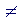0 -- upon dividing f(x) by x − 7, then (x − 7) is not a factor of f(x).  And according to the factor theorem, 7 is not a root of f(x).

Problem 3.   Let  g(x) = 3x 4 + 17x3 + 16x 2 − 10x + 4.  Use synthetic division to divide g(x) by x + 2.According to the remainder theorem, g(−2) = 0.

Therefore, what do you conclude about −2?

−2 is a root of g(x).

What do you conclude about (x + 2)?

(x + 2) is a factor of g(x).

Problem 4.   Use synthetic division to divide

x3 + 125   by  x + 5.x3 + 125 = (x2 − 5x + 25)(x + 5)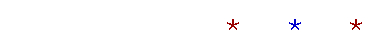Next Topic:  Roots of polynomials

Please make a donation to keep TheMathPage online.
Even \$1 will help.